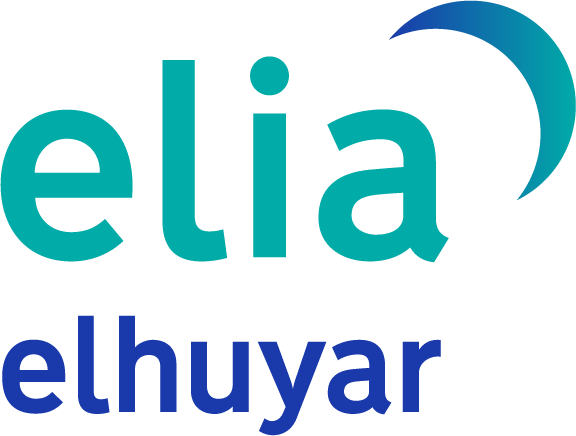}

# Resolution of programs based on conversations

1986/12/01 Arrojeria, Eustakio - Elhuyar Zientziaren Komunikazioa | Lizaso, Pili - Informatika SailaElhuyar Fundazioa Iturria: Elhuyar aldizkaria

The resolution of the programme here is quite simple and understandable.

Programs in which conversations are of great importance are numerous in teaching. And today, this program is one of them. On the other hand, when making this explanation, it has not been intended to respond accurately to this type of programs (although for this it is based on a specific case), but to show how you can act before such programs.

Therefore, and like you, reader, you can confirm it, the resolution of the program here is quite simple and comprehensive. The computer will ask for radio and height if necessary. Then, and according to given orders, you will calculate the volume of a sphere, cone or cylinder indicating the result.

As a clarification, the organizational chart of this program is as follows:

INTERVIEW PROGRAM 10 REM ** 20 CLS 30 LET PI=3.141592 40 PRINT "This program calculates the volumes of spheres, cones or cylinders" 50 FOR T = 1 TO 1200 60 I = I + 1 70 NEXT T 80 CLS 90 What type of PRINT do you want to calculate 100? 110 PRINT 120 PRINT "If sphere, number 1" 130 PRINT "If cone, put 2" 140 PRINT "If cylinder, put 3" 150 INPUT A 160 IF A = 1 THEN GOSUB 200: GOTO 430 170 IF A = 2 THEN GOSUB 270: GOTO 430 180 IF A = 3 THEN GOSUB 350: GOTO 430 190 GOTO 80 200 REM ** Sphere volume ** 210 CLS: PRINT "The sphere volume is: V = 4 * PI * R> 3/3" 220 INPUT "How much the sphere radius is worth"; R 230 LET V = 4 * PI * R> 3/3 240 PRINT "Sphere volume: V ="; V 250 RETURN 260 REM ** End of sphere volume ** 270 REM ** Cone volume ** 280 CLS: PRINT "Cone volume is: V = PI * R\ 2 * H/3" 290 INPUT "How much the radius of the cone foot is worth"; R 300 INPUT "And the cone height"; H 310 LET V = PI * R< 2 * H/3 320 PRINT "Cone volume: V ="; V 330 RETURN 340 REM ** End of cone volume ** 350 REM ** Cylinder volume ** 360 CLS: PRINT "Cylinder volume is: V = PI * R> 2 * H" 370 INPUT "How much the cylinder foot radius is worth"; R 380 INPUT "And the cylinder height"; H 390 LET V = PI * R< 2 * H 400 PRINT "Cylinder volume: V ="; V 410 RETURN 420 REM ** End of cylinder volume ** 430 INPUT "Want to calculate another volume (SI/NO)"; C$440 IF C$ = "SI" OR C$= " THEN GOTO 20 450 IF PRINT$ 470 NO Similar program 500 NDTeu es fr en ca gl

## Gai honi buruzko eduki gehiago

Elhuyarrek garatutako teknologia